# Bihar Board Class 9th Maths Solutions Chapter 12 Heron’s Formula Ex 12.1

Bihar Board Class 9th Maths Solutions Chapter 12 Heron’s Formula Ex 12.1 Textbook Questions and Answers.

## BSEB Bihar Board Class 9th Maths Solutions Chapter 12 Heron’s Formula Ex 12.1Question 1.
A traffic signal board, indicating ‘SCHOOL AHEAD’, is an equilateral triangle with side ‘a’. Find the area of the signal board, using Heron’s formula. If its perimeter is 180 cm, what will be the area of the signal board ?
Solution:
To find the area of an equilateral triangle using Heron’s formula :
If a, b, c be the lengths of sides BC, CA and AB of ∆ ABC and if s = $$\frac { 1 }{ 2 }$$(a + b + c), then
area of ∆ ABC = $$\sqrt{s(s-a)(s-b)(s-c)}$$
Since ∆ ABCis given as equilateral of side = a
∴ Here a = b = c (= a) and s = $$\frac { 1 }{ 2 }$$(a + a + a) = $$\frac { 3a }{ 2 }$$To find the area, when its perimeter = 180 cm
Here a + a + a – 180 cm
⇒ 3a = 180 cm
⇒ a = 60 cmQuestion 2.
The triangular side walls of a flyover have been used for advertisements. The sides of the walls are 122 m, 22 m and 120 m (see figure). The advertisements yield an earning of Rs 5000 per m2 per year. A company hired one of its walls for 3 months. How much rent did it pay?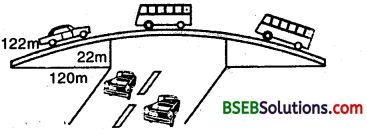Solution:
First we find the area of triangular side measuring 122 m, 22 m and 120 m.
Let a = 122 m, b = 22 m and c = 120 m.
∴ s = $$\frac { 1 }{ 2 }$$(a + b + c) = $$\frac { 1 }{ 2 }$$(122 + 22 + 120) m
= ( $$\frac { 1 }{ 2 }$$ x 264) m = 132 m
Now,
s – a = (132 – 122) m = 10 m
s – b = (132 – 22) m = 110 m
and
s – c = (132 – 120) m = 12 m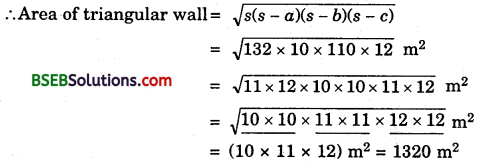Rent charges = Rs 5000 per m² per year.
∴ Rent charged from a company for 3 months
= Rs (5000 x 1320 x $$\frac { 3 }{ 12 }$$)
= Rs, 16,50,000Question 3.
There is a slide in a park. One of its side walls has been painted in blue colour with a message “KEEP THE PARK GREEN AND CLEAN”, (See figure). If the sides of the wall are 15 m, 11 m and 6 m, find the area painted in colour.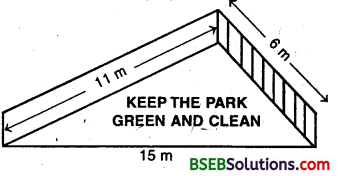Solution:
First find the area of side wall measuring 15 m, 11 m and 6 m.
Let a = 15 m, b = 11 m, c = 6 m
and s = $$\frac { 1 }{ 2 }$$ (a + b + c) = $$\frac { 1 }{ 2 }$$ (15 + 11 + 6) m
= ($$\frac { 1 }{ 2 }$$ x 32) m = 16 m
Now,
s – a = (16 – 15) m = 1 m
s – b = (16 – 11) m = 5 m
and
s – c = (16 – 6) m = 10 m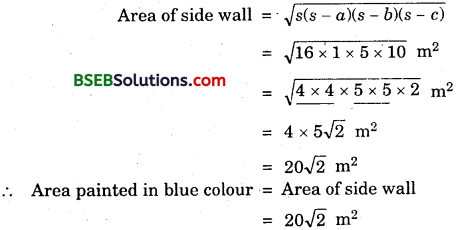Question 4.
Find the area of a triangle two sides of which are 18 cm and 10 cm and the perimeter is 42 cm.
Solution:
Let a, b and c be the sides of a triangle such that
a = 18 cm, b = 10 cm and a + b + c = 42 cm.
∴ c = 42 – a – b
⇒ c = (42 – 18 – 10) cm = 14 cm
Now, s = $$\frac { 1 }{ 2 }$$(a + b + c) = $$\frac { 1 }{ 2 }$$ x 42 cm = 21 cm
s – a = (21 – 18) cm = 3 cm
s – b = (21 – 10) cm = 11 cm
and s – c = (21 – 14) cm = 7 cmQuestion 5.
Sides of a triangle are in the ratio of 12 : 17 : 25 and its perimeter is 540 cm. Find its area.
Solution:
Let the sides be a, b and c of the triangle.
∴ a : b : c = 12 : 17 : 25 a b c
⇒ $$\frac { a }{ 12 }$$ = $$\frac { b }{ 12 }$$ = $$\frac { c }{ 25 }$$ = k(Say)
⇒ a = 12k, b = 17k and c = 25k
Also, perimeter = 540 cm
⇒ a + b + c = 540
⇒ 12k + 17k + 25k = 540
⇒ 54k = 540 i.e., k = 10
Thus, a = 12 x 10 = 120 cm, b = 17 x 10 cm = 170 and c = 25 x 10 = 250 cm.
s = $$\frac { 1 }{ 2 }$$ x 540 cm = 270 cm
s – a = (270 – 120) cm = 150 cm
s-b = (270 – 170) cm = 100 cm
and
s – c = (270 – 250) cm = 20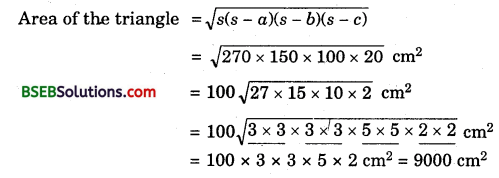Question 6.
An isosceles triangle has perimeter 30 cm and each of the equal sides is 12 cm. Find the area of the triangle.
Solution:
Here a = b = 12 cmand a + b + c = perimeter = 30 cm
⇒ c = (30 – a – b) cm
⇒ c = (30 – 12 – 12) cm = 6 cm
Now, s = $$\frac { 1 }{ 2 }$$ x 30 cm = 15 cm
s – a = (15 – 12) cm = 3 cm,
s – b = (15 – 12) cm = 3 cm
and
s – c = (15 – 6) cm = 9 cm